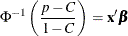Response Level Ordering

For binary response data, PROC PROBIT fits the following model by default:where p is the probability of the response level identified as the first level in the Response Profile table in the output andis the normal cumulative distribution function. By default, the covariate vector x contains an intercept term. This is sometimes called Abbot’s formula.

Because of the symmetry of the normal (and logistic) distribution, the effect of reversing the order of the two response values is to change the signs ofin the preceding equation.

By default, response levels appear in ascending, sorted order (that is, the lowest level appears first, and then the next lowest, and so on). There are a number of ways that you can control the sort order of the response categories and, therefore, which level is assigned the first ordered level. One of the most common sets of response levels is {0,1}, with 1 representing the event with the probability that is to be modeled.

Consider the example where Y takes the values 1 and 0 for event and nonevent, respectively, and EXPOSURE is the explanatory variable. By default, PROC PROBIT assigns the first ordered level to response level 0, causing the probability of the nonevent to be modeled. There are several ways to change this.

Besides recoding the variable Y, you can do the following:

• assign a format to Y such that the first formatted value (when the formatted values are put in sorted order) corresponds to the event. For the following example, Y=0 could be assigned formatted value ‘nonevent’ and Y=1 could be assigned formatted value ‘event.’ Since ORDER=FORMATTED by default, Y=1 becomes the first ordered level. See Example 75.3 for an illustration of this method.

proc format;
value disease 1='event' 0='nonevent';
run;
proc probit;
model y=exposure;
format y disease.;
run;

• arrange the input data set so that Y=1 appears first and use the ORDER=DATA option in the PROC PROBIT statement. Since ORDER=DATA sorts levels in order of their appearance in the data set, Y=1 becomes the first ordered level. Note that this option causes classification variables to be sorted by their order of appearance in the data set, also.